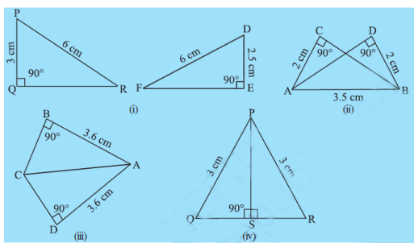# 1.  In Fig 7.32, measures of some parts of triangles are given.By applying RHS congruence rule, state which pairs of triangles are congruent. In case of congruent triangles, write the result in symbolic form.P Pankaj Sanodiya

i) In  and

Hence they are not congruent.

ii)

In  and

( same side )

So, by RHS congruency rule,

iii)

In  and

( same side )

So, by RHS congruency rule,

iv)

In  and

( same side )

So, by RHS congruency rule,

Exams
Articles
Questions